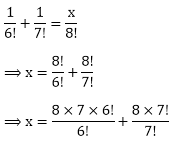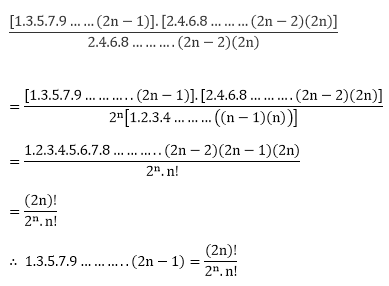×#### Thank you for registering.

One of our academic counsellors will contact you within 1 working day.

Click to Chat

1800-1023-196

+91-120-4616500

CART 0

• 0

MY CART (5)

Use Coupon: CART20 and get 20% off on all online Study Material

ITEM
DETAILS
MRP
DISCOUNT
FINAL PRICE
Total Price: Rs.

There are no items in this cart.
Continue Shopping• Complete JEE Main/Advanced Course and Test Series
• OFFERED PRICE: Rs. 15,900
• View Details

```Chapter 16: Permutations – Exercise 16.1

Permutations – Exercise – 16.1 – Q.1

We have,= 30 × 29

= 870(ii) We have= 10 × 10

= 100

(iii) We have,

8! = 8 × 7 × 6 × 5 × 4 × 3 × 2 × 1

7! = 7 × 6 × 5 × 4 × 3 × 2 × 1

and 6! = 6 × 5 × 4 × 3 × 2 × 1

∴  L. C. M. (6!, 7!, 8!) = 8!

Permutations – Exercise – 16.1 – Q.2

L.H.S:Permutations – Exercise – 16.1 – Q.3(i)

We have,⟹ x = 6 × 6

⟹ x = 36.

Hence, x = 36.

Permutations – Exercise – 16.1 – Q.3(ii)⟹ x = 10 × 9 + 10

⟹ x = 100

Permutations – Exercise – 16.1 – Q.3(iii)⟹ x = 8 × 7 + 8

⟹ x = 64

Permutations – Exercise – 16.1 – Q.4(i)

We have,

5 × 6 × 7 × 8 × 9 × 10Permutations – Exercise – 16.1 – Q.4(ii)

We have,

3 × 6 × 9 × 12 × 15 × 18

= 3 ×(3 × 2) × (3 × 3) × (3 × 4) × (3 × 5) × (3 × 6)

= 36 × [2 × 3 × 4 × 5 × 6]

= 36 × (6!)

Permutations – Exercise – 16.1 – Q.4(iii)

We have,

(n + 1)(n + 2)(n + 3)…………(2n)

= [1 × 2 × 3 × 4………..(n - 1)n] × (n + 1)(n + 2) .. (2n - 1) × 2n

[1 × 2 × 3 × 4………(n - 1)n]

= (2n!)!/n!

Permutations – Exercise – 16.1 – Q.4(iv)

We have,

1 × 5 × 7 × 9………….. (2n - 1)Permutations – Exercise – 16.1 – Q.5

(i) LHS = (2 + 3)!

= 5!

= 5 × 4 × 3 × 2 × 1

= 120

and, RHS = 2! + 3!

= 2 × 1 + 3 × 2

= 2 × 1 + 3 × 2 × 1

= 2 + 6

= 8

∴ 120 ≠ 8

∴ (2 + 3)! ≠ 2! + 3!

So, it is false.

(ii) LHS = (2 × 3)!

= 6!

= 6 × 5 × 4 × 3 × 2 × 1

= 720

and, RHS = 2! × 3!

∴ 720 ≠ 12

∴ (2 × 3)! ≠ 2! × 3!

Hence, it is false.

Permutations – Exercise – 16.1 – Q.6

LHS = n! + (n + 1)!

= n! + (n + 1)(n + 1 - 1)!

= n! + (n + 1)n!

= n!(1 + n + 1)

= n!(n + 2)

= LHS

∴  n!(n + 2) = n! + (n + 1)!

Hence, proved

Permutations – Exercise – 16.1 – Q.7

We have,

(n + 2)! = 60[(n - 1)!]

(n + 2)(n + 1)(n)(n - 1)! = 60[(n - 1)!]

⟹ (n + 2)(n + 1)n = 60

⟹ (n + 2)(n + 1)n = 5 × 4 × 3

∴ n = 3             [By comparing]

Hence, n = 3

Permutations – Exercise – 16.1 – Q.8

We have,

(n + 1)! = 90[(n - 1)!]

⟹ (n + 1) × n × (n - 1)! = 90[(n - 1)!]

⟹ n(n + 1) = 90

⟹ n2 + n = 90

⟹ n2 + n - 90 = 0

⟹ n2 + n - 90 = 0

⟹ n2 + n - 90 = 0

⟹ n2 + 10n - 9n - 90 = 0

⟹ n(n + 10)(n + 10) = 0

⟹ n - 9 = 0                  [∵ n + 10 ≠ 0]

⟹ n = 9

Hence, n = 9

```### Course Features

• 728 Video Lectures
• Revision Notes
• Previous Year Papers
• Mind Map
• Study Planner
• NCERT Solutions
• Discussion Forum
• Test paper with Video Solution